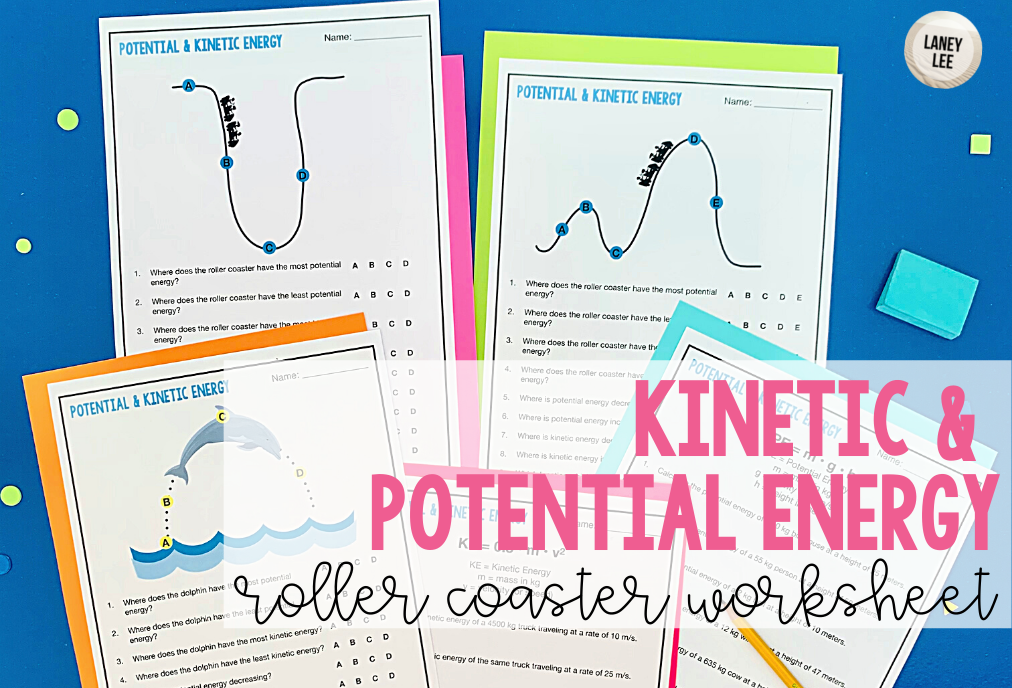## Potential Energy and Kinetic Energy Roller Coaster WorksheetLearn physics with this Kinetic and potential energy roller coaster worksheet. This resource includes 5 pages of student practice. There are three pages where students identify points (highest amount of kinetic energy, potential energy is decreasing, etc.) and two pages where students will solve using the kinetic and potential energy equations.